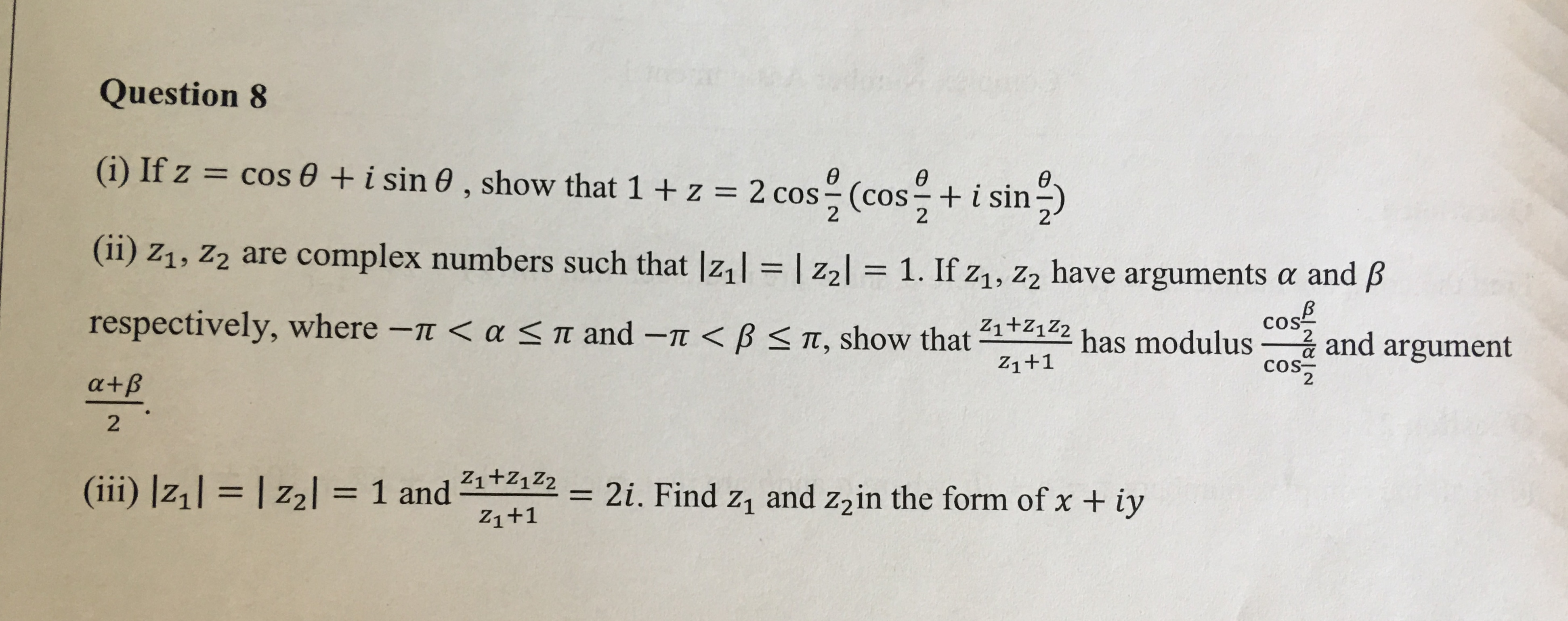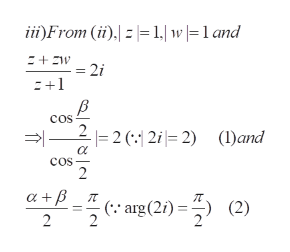# Question 8(i) If z cos 0 + i sin 0 , show that 1 +z = 2 cos (cOS+ i sin)өө2(ii) z1, Z2 are complex numbers such that Iz,1l | z 2l = 1. If z1, Z2 have argumentsa and Bcos2respectively, where -T < a< m and -n

Question

Hi, the question is attached!

I only need part iii), so don't worry about i) and ii) !!!!

Thanks ?help_outlineImage TranscriptioncloseQuestion 8 (i) If z cos 0 + i sin 0 , show that 1 +z = 2 cos (cOS+ i sin) ө ө 2 (ii) z1, Z2 are complex numbers such that Iz,1l | z 2l = 1. If z1, Z2 have arguments a and B cos 2 respectively, where -T < a < m and -n
check_circle

Step 1

To determine the complex numbers z and w (change notation to avoid suffixes) satisfying the given conditions

Step 2

From (ii), set up equations f...help_outlineImage Transcriptioncloseii)From () 1.| w |=1 and = 2i 1 cos 2 2 (:1 2i 2) (1)and - cos 2 arg(2)=(2) 2 fullscreen

### Want to see the full answer?

See Solution

#### Want to see this answer and more?

Solutions are written by subject experts who are available 24/7. Questions are typically answered within 1 hour.*

See Solution
*Response times may vary by subject and question.
Tagged in

### Math